# Arithmetical Proportion

To put in a general form what Macdonald has described in his introduction “Ratios and Proportions”, an arithmetical proportion is of the form a-b=c-d. The terms a and c are called antecedents and the terms b and d are called consequents. Further, the terms a and d are called the extremes and the terms b and c are called the means.

Macdonald provides six rules for arithmetical proportions. He illustrates the rules with numbers; I will do it with symbols.

1. Adding a constant to each arithmetical ratio maintains an arithmetical proportion (for any constant k,k+a-b=k+c-d).
2. Multiplying each arithmetical ratio by a constant maintains an arithmetical proportion (for any constant k,k(a-b)=k(c-d)).
3. The sum of the means is equal to the sum of the extremes (a+b=b+c).
4. Transferring the two means or transferring the two extremes does not destroy the equality, i.e. it maintains an arithmetical proportion (transferring the means: a-c=b-d; transferring the extremes d-b=c-a).
5. Several arithmetical proportions added together is also an arithmetical proportion.

(If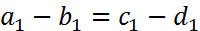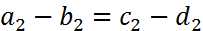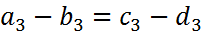then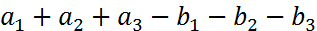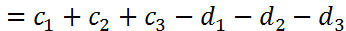)

1. Given three of the numbers in an arithmetical proportion the fourth can be determined, in Macdonald’s words, by adding the means and subtracting and extracting the one extreme from the result (if a-b=c-x then x= (b+c)-a).

Then Macdonald introduces the idea of an arithmetical progression which is treated later in his notebook. When in an arithmetical proportion, the same number which has been the consequent in the first ratio is the antecedent in the second ratio, the proportion is called a continued arithmetical proportion (a-b=b-c). When there is a series of continued arithmetical proportions (a-b=b-c=c-d=d-e=e-f=f-g) the distinct terms in the arithmetical ratios form an arithmetical progression (a, b, c, d, e, f, g are an arithmetical progression).